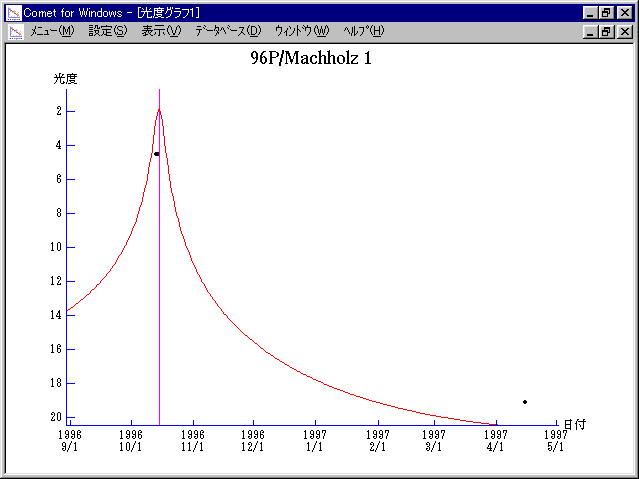# \$B%^%C%/%[%k%DBh#1WB@1(B

96P/Machholz 1 (1996)###\$B50F;MWAG(B

```   The following improved orbital elements, by Kenji Muraoka, are
from 105 observations 1986 to 1997, perturbations by 9 Planets,
Moon and 5 minor planets were taken into account.
The mean residual is +/- 0.94 arc seconds.

Epoch  =  1996 Oct.  4.0  TT       JDT = 2450360.5
T  =  1996 Oct. 15.06955       +/- 0.00003 (m.e.) TT
Peri. =   14.58607                +/- 0.00004
Node  =   94.53202                +/- 0.00003   (2000.0)
Incl. =   60.07419                +/- 0.00008
q  =    0.1247178              +/- 0.0000003 AU
e  =    0.9586366              +/- 0.0000001
a  =    3.0151757              +/- 0.0000037 AU
n  =    0.18825007             +/- 0.00000034
P  =    5.236                  +/- 0.0000095  years
```

###\$B@1?^(B1996\$BG/(B 6\$B7n(B 6\$BF|!A(B1997\$BG/(B 2\$B7n(B11\$BF|(B

###\$B8wEYJQ2=(B

```        m1 = 12.7 + 5 log\$B&\$(B + 12.0 log r
```##### \$B50F;MWAG\$OB<2,7r<#;a\$N7W;;\$K\$h\$k\$b\$N\$G\$9!#(B \$B@1?^\$O(B StellaNavigator Ver.2.0 for Windows (\$B%"%9%H%m%"!<%D(B \$BJTCx(B / \$B%"%9%-!<=PHG6I4)(B) \$B\$G:n@.\$7\$?\$b\$N\$G\$9!#(B \$B8wEY%0%i%U\$O(BComet for Windows\$B\$G:n@.\$7\$?\$b\$N\$G\$9!#(B